# Cuboid

How many times will increase the volume of a cuboid, if one dimension is twice larger, second dimension three times larger and third dimension four times lower?

n =  1.5

### Step-by-step explanation: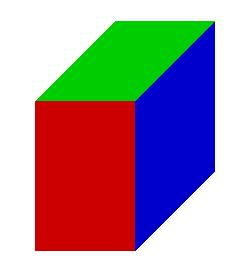Did you find an error or inaccuracy? Feel free to write us. Thank you!Tips to related online calculators
Tip: Our volume units converter will help you with the conversion of volume units.

## Related math problems and questions:

• Cube 3How many times will increase the volume of a cube if we double the length of its edge?
• Third dimensionCalculate the third dimension of the cuboid: a) V = 224 m3, a = 7 m, b = 4 m b) V = 216 dm3, a = 9 dm, c = 4 dm
• Cuboid aquariumCuboid 25 times 30 cm. How long is third side if cuboid contains 30 liters of water?
• Cube 2How many times will increase if the surface area of the cube if we triple length of its edge?
• Cubes into cuboidHow many 12 centimeter cubes fit into the block (cuboid) with 6dm, 8.4dm, and 4.8?
• CubeOne cube has an edge increased five times. How many times will larger it's surface area and volume?
• Cuboid - VabFind the surface of the cuboid when its volume is 52.8 cubic centimeters, and the length of its two edges is 2 centimeters and 6 centimeters.
• Cuboid to cubeA cuboid with dimensions of 9 cm, 6 cm, and 4 cm has the same volume as a cube. Calculate the surface of this cube.
• Trapezium zoomHow many times increase area of trapezoid if all sides and altitude increase 5 times?
• Surface and volumeFind the surface and volume of a cuboid whose dimensions are 1 m, 50 cm, and 6 dm.
• Fe vs. H2O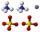The volume of what the body of the same weight is greater - iron or water?
• Volume of sphereHow many times does the volume of a sphere increase if its radius increases 2 ×?
• AquariumCan 30 liters of water fit in a cuboid aquarium with dimensions a = 3dm b = 6dm c = 5dm?
• Milk cartonsHow much paper do we need for 12 tetra packs with dimensions 6 cm, 11 cm, and 20 cm? Will 1 liter of milk fit in the box?
• The wall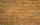We have to build a cuboid wall with dimensions base 30 cm and 45 cm and height 3.25 meters. Calculate how many we need bricks if we spend 400 pieces of bricks to 1 m3 of wall?
• Mike chose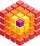Mike chose 4 identical cubes, 3 identical prisms and 2 identical cylinders from the kit. The edge of the cube is 3 cm long. The prism has two dimensions the same as the cube, its third dimension is 2 times longer. The diameter of the base of the cylinder
• Edges of the cuboid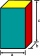Find the length of the edges of the cuboid, which has the following dimensions: width is 0.4 m; the height is 5.8 dm and the block can hold 81.2 liters of fluid.